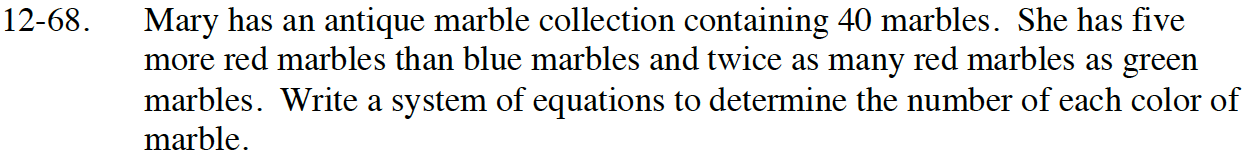### Home > INT3 > Chapter Ch12 > Lesson 12.1.4 > Problem12-68

12-68.

Mary has an antique marble collection containing 40 marbles. She has five more red marbles than blue marbles and twice as many red marbles as green marbles. Write a system of equations to determine the number of each color of marble. Homework Help ✎Let b = the number of blue marbles, r = the number of red marbles,
and g = the number of green marbles.

b + 5 = r
2g = r
r + g + b = 40

Solve the system of equations using a combination of substitution and elimination.